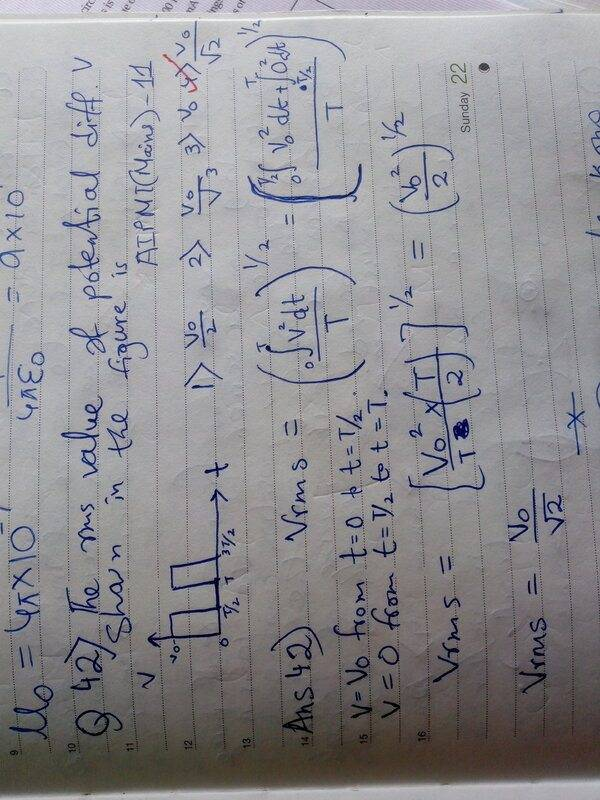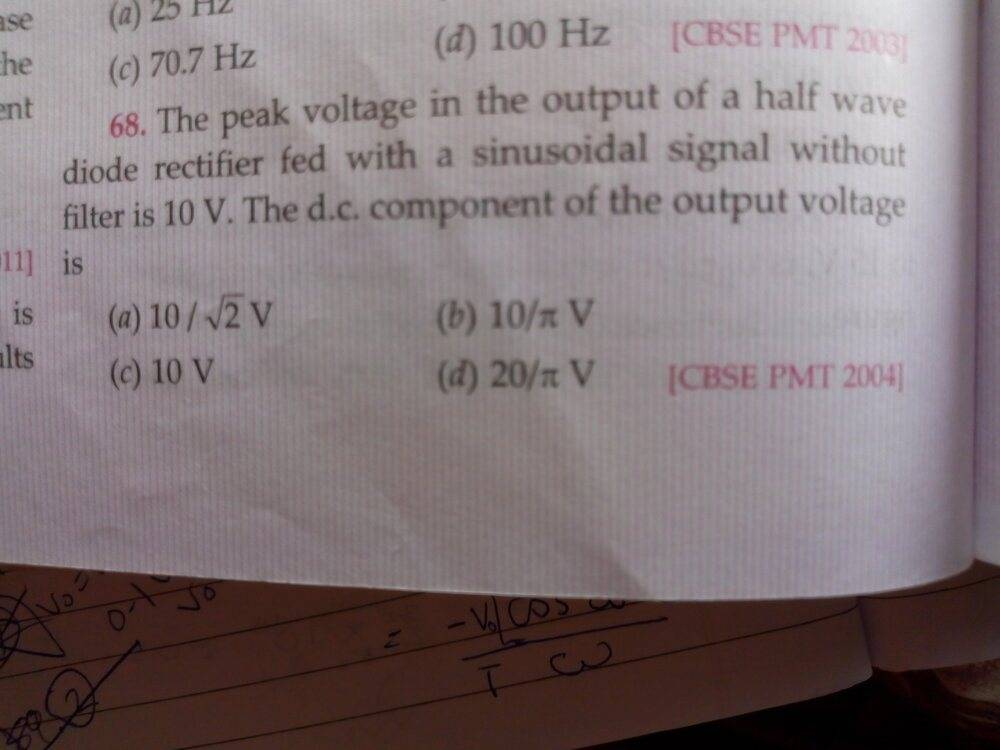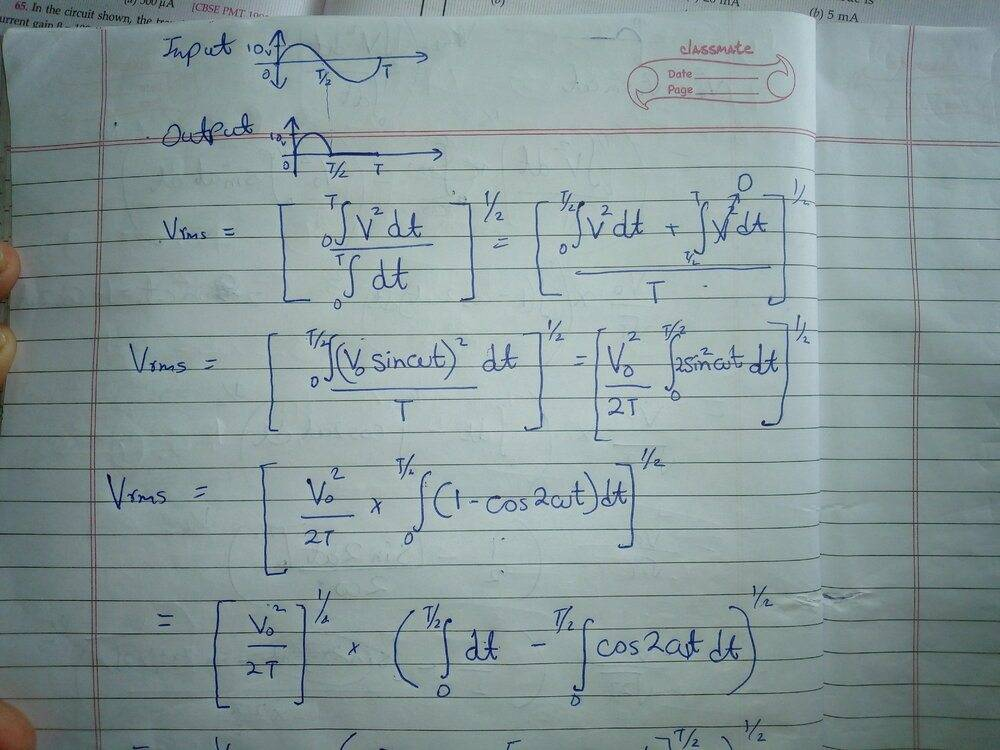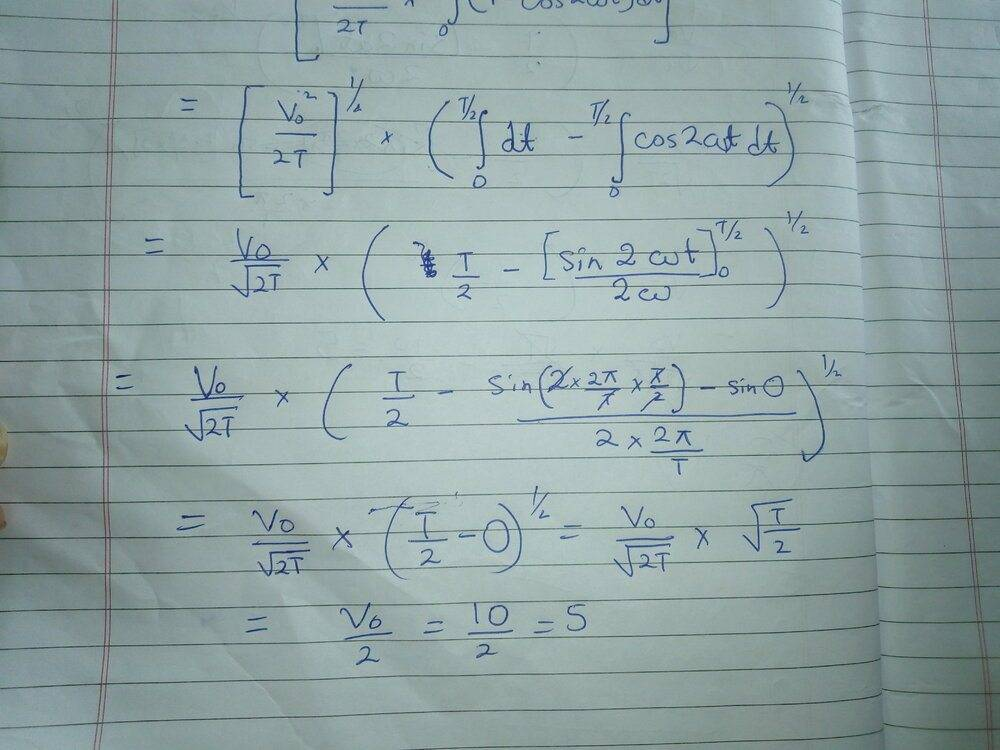# RMS value

Rongeet Banerjee
Homework Statement:
How can I find the RMS value of a DC Current?
Relevant Equations:
Vrms=Vpeak/(2)½I had previously solved this using Root Mean Square method by integrating the value of voltage from t=0 to t=T/2 and then from t=T/2 to t=T.Answer was Vo/2½.Yesterday I found this question👇🏾if I followed the previous approach then:5 volts is not even in the option. How can I find the DC component of an Output Sinusoidal Voltage?

Homework Helper
Gold Member
Homework Statement:: How can I find the RMS value of a DC Current?
Relevant Equations:: Vrms=Vpeak/(2)½

I had previously solved this using Root Mean Square method
This gives you the dc "equivalent" of the time varying signal, not the dc "component" of it.

Rongeet Banerjee
Coming back to question 42,how can we find RMS value of a non alternating current?

archaic
For DC, the actual value is the RMS value.

Homework Helper
Gold Member
Coming back to question 42,how can we find RMS value of a non alternating current?
What is question 42?
Edit: Ok I can see that in your first image. Haven't you already solved it correctly? It is a non-alternating waveform. What else do you want to know?

Ok.But isn't the average value of AC 2Vpeak/π.
No. The question mentions "a half wave" rectifier. What will the output voltage look like?

Also, ac in electricity usually means a periodic signal with both positive and negative halves (mostly sinusoidal signals). So the average value of ac is 0.

Rongeet Banerjee
This gives you the dc "equivalent" of the time varying signal, not the dc "component" of it.
So basically when the question asked me to find RMS value they actually meant"DC equivalent".I got a bit confused because I had learned that only alternating currents have RMS values.So in reality is RMS value used for any fluctuating physical quantity?

Rongeet Banerjee
DC Component =(average of V from t=0 to t=T/2 + average of V fom t=T/2 to t=T) /2 i.e. =(20/π + 0)/2 =10/π .Is that the answer?

Homework Helper
Gold Member
So in reality is RMS value used for any fluctuating physical quantity?
Yes, if they are fluctuating periodically.
Same is true for average value.
You compute rms and average values of a periodic signal and derive conclusions from it.
If the average of a periodic signal is 0, it is symmetric about the time axis (i.e. equal positive and negative parts).
If the average is non zero, it has a dc "component" in it which is making the positive and negative parts unequal.

If you are passing an alternating current through a resistor, the heat produced by the alternating current in its one cycle time is equal to the heat produced by its rms current(dc) in the same time. This means the rms current is the dc "equivalent" of the alternating current.

•Rongeet Banerjee
Homework Helper
Gold Member
DC Component =(average of V from t=0 to t=T/2 + average of V fom t=T/2 to t=T) /2 i.e. =(20/π + 0)/2 =10/π .Is that the answer?
Yes that is the answer, but I am not sure if the formula you wrote is correct. Is that some "trick" or "shortcut" considering the MCQ nature of some exam?
I prefer the general formula:
Average= integral of function/time period.
You can then split the integral as per the function behavior between t=0 to t=T.

In this case, your function is sinusoidal from 0 to T/2 and 0 from T/2 to T. You can split the integral accordingly and get the average value.